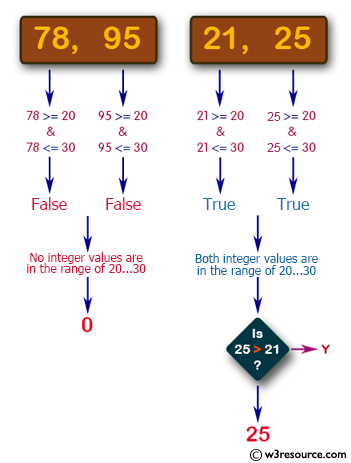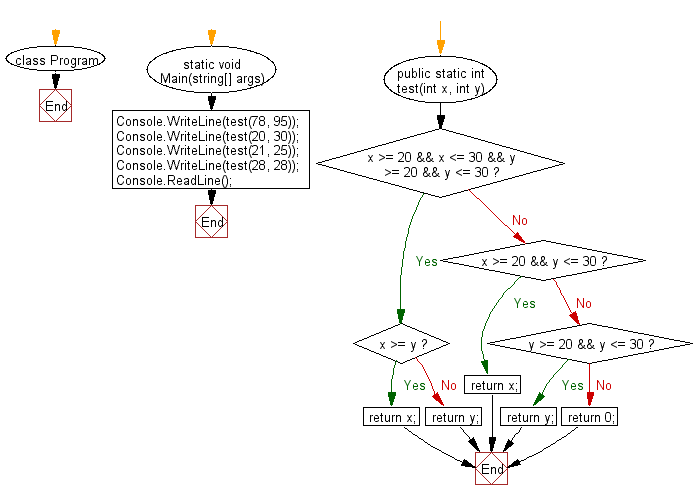﻿ C# - Larger from two positive values in the range 20..30# C# Sharp Basic Algorithm Exercises: Find the larger value from two positive integer values that is in the range 20..30 inclusive

## C# Sharp Basic Algorithm: Exercise-21 with Solution

Write a C# Sharp program to find the larger value from two positive integer values that is in the range 20..30 inclusive, or return 0 if neither is in that range.

Pictorial Presentation:Sample Solution:-

C# Sharp Code:

``````using System;
namespace exercises
{
class Program
{
static void Main(string[] args)
{
Console.WriteLine(test(78, 95));
Console.WriteLine(test(20, 30));
Console.WriteLine(test(21, 25));
Console.WriteLine(test(28, 28));
}

public static int test(int x, int y)
{
if (x >= 20 && x <= 30 && y >= 20 && y <= 30)
{
if (x >= y)
{
return x;
}
else
{
return y;
}
}
else if (x >= 20 && y <= 30)
{
return x;
}
else if (y >= 20 && y <= 30)
{
return y;
}
else
{
return 0;
}
}
}
}
```
```

Sample Output:

```0
30
25
28```

Flowchart:C# Sharp Code Editor:

Improve this sample solution and post your code through Disqus

What is the difficulty level of this exercise?

Test your Programming skills with w3resource's quiz.

﻿